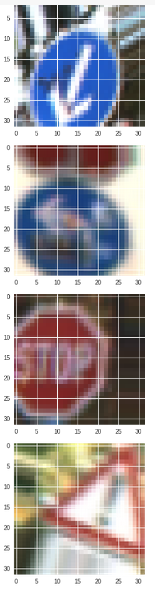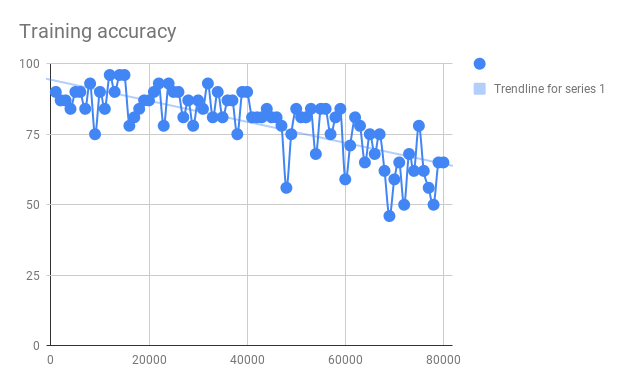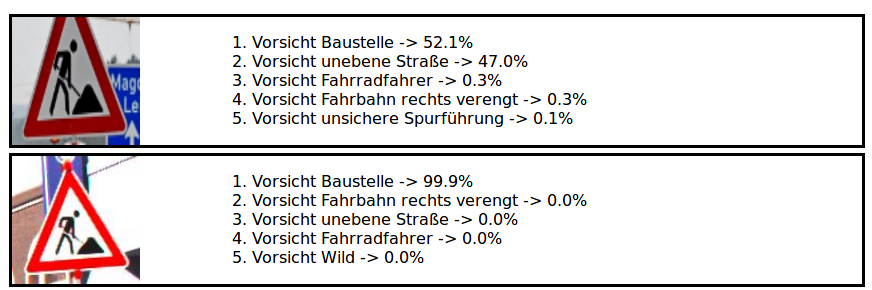# Image data set augmentation

by Daniel Pollithy

## Transform images to increase the size of the GTSRB dataset

My GTSRB Keras CNN is overfitting its training data. I know that because the accuracy on the training data ist above 98% but the accuracy on the test data was 84% for its best run.

Before I balanced the classes of the data set, the test accuracy was even lower (80%). Adding L2 regularization to the last dense layer added 1%.

A gap of more than 10% remained, so I tried other strategies. Increasing the batch_size did not help. Doubling the size of the images (32x32 -> 64x64) had no certain effect.

But I kept the best option for the end: Data set augmentation. For images the idea is to transform the images randomly (shifting the pixels, rotating, zooming…). This is intuitively a really important building block in the training pipeline in order to avoid that the model finds “too simple” methods like checking whether a pixel at an exact position is white. Although the convolutional layers and pooling layers already help a little bit here.

## Code

I am using the same Keras code as in my last blog entries for the exact same dataset.

I only have to add an ImageDataGenerator:

``````
from keras.preprocessing.image import ImageDataGenerator

datagen = ImageDataGenerator(
#featurewise_center=True,
#featurewise_std_normalization=True,

#samplewise_center=True,
#samplewise_std_normalization=True,

rotation_range=20,
width_shift_range=0.2,
height_shift_range=0.2,
horizontal_flip=False,
zoom_range=0.2,
shear_range=15
)

# compute quantities required for featurewise normalization
# (std, mean, and principal components if ZCA whitening is applied)
datagen.fit(X_balanced)

``````

And replace the training control:

``````
%matplotlib inline

from keras.callbacks import LearningRateScheduler, ModelCheckpoint

def lr_schedule(epoch):
return lr * (0.1 ** int(epoch / epochs))

epochs = 10
batch_size = 32

# here's a more "manual" example
for e in range(epochs):
print('Epoch', e)
batches = 0
for x_batch, y_batch in datagen.flow(X_balanced, Y_balanced, batch_size=batch_size):
verbose = batches % 1000 == 0

# show some images
if verbose:
for i in range (0, batch_size):
image = x_batch[i]
plt.imshow(image)
plt.show()

model.fit(x_batch, y_batch, verbose=verbose,
callbacks=[LearningRateScheduler(lr_schedule),
ModelCheckpoint('model.h5', save_best_only=True)])
batches += 1
if batches >= len(X_balanced) / epochs:
# we need to break the loop by hand because the generator loops indefinitely
break
``````The transformed images are classifiable but it is getting harder… Let’s see whether this has an impact on the generalization error.

I can already tell that the training takes a lot longer, that could be because the ImageDataGenerator works on the CPU.

## Result

The training took 40 minutes instead of 5. And the training accuracy went down over time…And the test accuracy was a little bit lower 84,4% instead of 84,8% (without augmentation).

The parameters have to be adjusted.

I fixed the learning rate to 0.1 now. No exponential decay. The next step is to be able to get a high training accuracy to see whether we can get the network to overfit the data generating function…

I manually early stopped the training with a loss of 0.8872 and a training accuracy of 97%, which is good.

And the test accuracy got boosted by a signifact factor: Test accuracy = 0.9583531274742676. That’s a plus of 10%.

That is really impressing. I am going to upload this new model into my tensorflow.js application.I can upload a badly cropped and skewed image now and it gets classified correctly. Wow! I am surprised how well that worked.